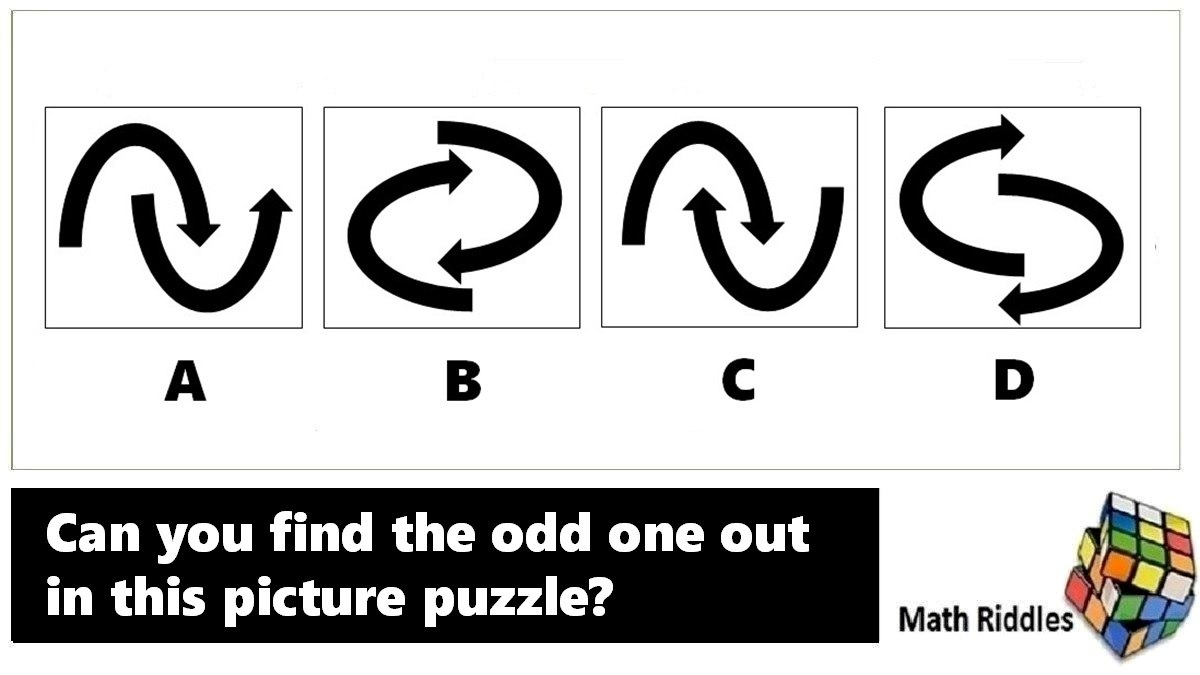# Math Riddles IQ Test: Find the Odd One Out Picture Puzzle

Math Riddles Challenge: Can you find the missing numbers in these math puzzles? Check Your IQ.Math Riddles IQ Test: Find the Odd One Out Picture Puzzle

Math Riddles IQ Test: Today, we bring a new set of math puzzles. Here we have two sets of picture puzzles where you have to find the odd one out. These picture puzzles require analytical reasoning. There are four images and one of them is the odd one out. Let us solve this fun series.

## Solution

### Picture Puzzle #1

When you turn Shape A anticlockwise 90 degrees, it matches Shape B.

When you turn Shape A clockwise 90 degrees, it matches Shape C.

Hence, A = B = C which leaves D as the odd one out.

### Picture Puzzle #2

When you rotate all the shapes by 180 degrees, all shapes match their original image in the question except for Shape A.

Hence, the odd-one-out image is Shape A.

### Picture Puzzle #3

Upon observation we notice,

In shape A, the outer figure has 4 sides and the inner figure has 3 sides.

In shape B, the outer figure has 5 sides and the inner figure has 4 sides.

In shape C, the outer figure has 4 sides and the inner figure has 4 sides as well.

In shape D, the outer figure has 7 sides and the inner figure has 6 sides.

Hence, we can conclude that except for Shape C, the number of sides of the outer figure is one more than the number of sides of the inner figure in all shapes.

Hence, the odd-one-out image is Shape C.

## Tell us in comments: Did you solve these math puzzles in 20 seconds each?

Check out more math puzzles!

Also Read: Math Riddles: Missing Number Series Puzzles, Solve in 20 Seconds Each

Also Read: Math Riddles: Missing Number Series Puzzles, Difficulty Level Hard